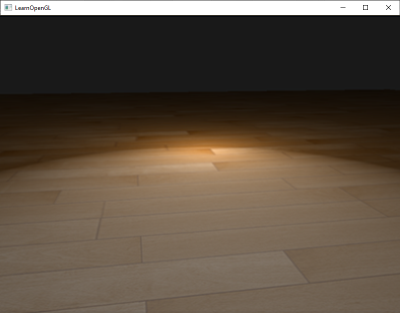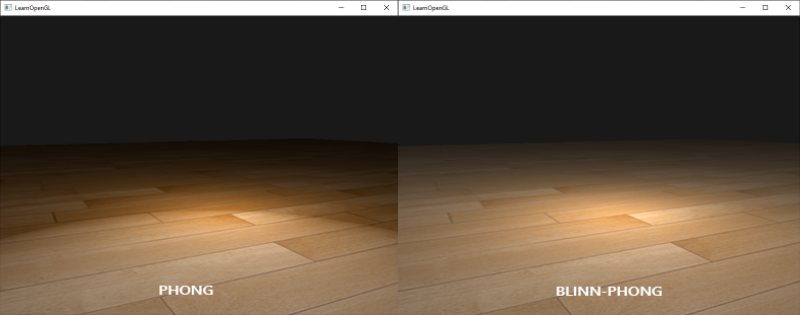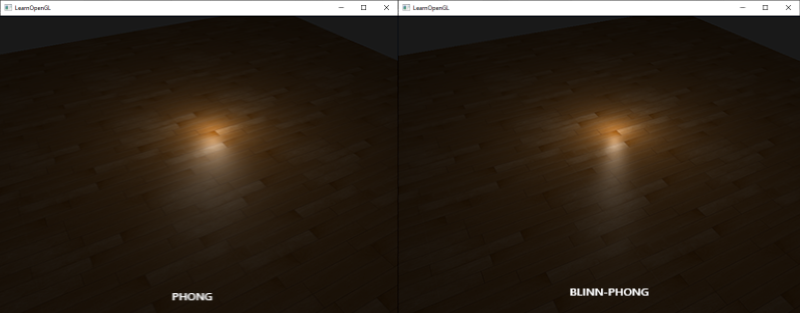If you're running AdBlock, please consider whitelisting this site if you'd like to support LearnOpenGL; and no worries, I won't be mad if you don't :)

In the lighting chapters we briefly introduced the Phong lighting model to bring a basic amount of realism into our scenes. The Phong model looks nice, but has a few nuances we'll focus on in this chapter.

## Blinn-Phong

Phong lighting is a great and very efficient approximation of lighting, but its specular reflections break down in certain conditions, specifically when the shininess property is low resulting in a large (rough) specular area. The image below shows what happens when we use a specular shininess exponent of 1.0 on a flat textured plane:You can see at the edges that the specular area is immediately cut off. The reason this happens is because the angle between the view and reflection vector doesn't go over 90 degrees. If the angle is larger than 90 degrees, the resulting dot product becomes negative and this results in a specular exponent of 0.0. You're probably thinking this won't be a problem since we shouldn't get any light with angles higher than 90 degrees anyways, right?

Wrong, this only applies to the diffuse component where an angle higher than 90 degrees between the normal and light source means the light source is below the lighted surface and thus the light's diffuse contribution should equal 0.0. However, with specular lighting we're not measuring the angle between the light source and the normal, but between the view and reflection vector. Take a look at the following two images:Here the issue should become apparent. The left image shows Phong reflections as familiar, with $$\theta$$ being less than 90 degrees. In the right image we can see that the angle $$\theta$$ between the view and reflection vector is larger than 90 degrees which as a result nullifies the specular contribution. This generally isn't a problem since the view direction is far from the reflection direction, but if we use a low specular exponent the specular radius is large enough to have a contribution under these conditions. Since we're nullifying this contribution at angles larger than 90 degrees we get the artifact as seen in the first image.

In 1977 the Blinn-Phong shading model was introduced by James F. Blinn as an extension to the Phong shading we've used so far. The Blinn-Phong model is largely similar, but approaches the specular model slightly different which as a result overcomes our problem. Instead of relying on a reflection vector we're using a so called halfway vector that is a unit vector exactly halfway between the view direction and the light direction. The closer this halfway vector aligns with the surface's normal vector, the higher the specular contribution.When the view direction is perfectly aligned with the (now imaginary) reflection vector, the halfway vector aligns perfectly with the normal vector. The closer the view direction is to the original reflection direction, the stronger the specular highlight.

Here you can see that whatever direction the viewer looks from, the angle between the halfway vector and the surface normal never exceeds 90 degrees (unless the light is far below the surface of course). The results are slightly different from Phong reflections, but generally more visually plausible, especially with low specular exponents. The Blinn-Phong shading model is also the exact shading model used in the earlier fixed function pipeline of OpenGL.

Getting the halfway vector is easy, we add the light's direction vector and view vector together and normalize the result:

$\bar{H} = \frac{\bar{L} + \bar{V}}{||\bar{L} + \bar{V}||}$

This translates to GLSL code as follows:


vec3 lightDir   = normalize(lightPos - FragPos);
vec3 viewDir    = normalize(viewPos - FragPos);
vec3 halfwayDir = normalize(lightDir + viewDir);


Then the actual calculation of the specular term becomes a clamped dot product between the surface normal and the halfway vector to get the cosine angle between them that we again raise to a specular shininess exponent:


float spec = pow(max(dot(normal, halfwayDir), 0.0), shininess);
vec3 specular = lightColor * spec;


And there is nothing more to Blinn-Phong than what we just described. The only difference between Blinn-Phong and Phong specular reflection is that we now measure the angle between the normal and halfway vector instead of the angle between the view and reflection vector.

With the introduction of the halfway vector we should no longer have the specular cutoff issue of Phong shading. The image below shows the specular area of both methods with a specular exponent of 0.5:Another subtle difference between Phong and Blinn-Phong shading is that the angle between the halfway vector and the surface normal is often shorter than the angle between the view and reflection vector. As a result, to get visuals similar to Phong shading the specular shininess exponent has to be set a bit higher. A general rule of thumb is to set it between 2 and 4 times the Phong shininess exponent.

Below is a comparison between both specular reflection models with the Phong exponent set to 8.0 and the Blinn-Phong component set to 32.0:You can see that the Blinn-Phong specular exponent is bit sharper compared to Phong. It usually requires a bit of tweaking to get similar results as to what you previously had with Phong shading. It's worth it though as Blinn-Phong shading is generally more realistic compared to default Phong shading.

Here we used a simple fragment shader that switches between regular Phong reflections and Blinn-Phong reflections:


void main()
{
[...]
float spec = 0.0;
if(blinn)
{
vec3 halfwayDir = normalize(lightDir + viewDir);
spec = pow(max(dot(normal, halfwayDir), 0.0), 16.0);
}
else
{
vec3 reflectDir = reflect(-lightDir, normal);
spec = pow(max(dot(viewDir, reflectDir), 0.0), 8.0);
}


You can find the source code for the simple demo here. By pressing the b key, the demo switches from Phong to Blinn-Phong lighting and vica versa.

HI